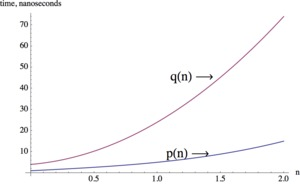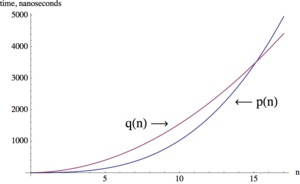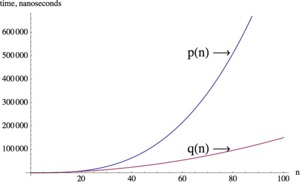# Running Time

Alignments to Content Standards: F-IF.C.7.c

Many computer applications use very complex mathematical algorithms. The faster the algorithm, the more smoothly the programs run. The running time of an algorithm depends on the total number of steps needed to complete the algorithm. For image processing, the running time of an algorithm increases as the size of the image increases.

For an $n$-by-$n$ image, algorithm 1 has running time given by $p(n) = n^3+3n+1$ and algorithm 2 has running time given by $q(n)=15n^2+5n+4$ (measured in nanoseconds, or $10^{-9}$ seconds ).

1. Compute the running time for both algorithms for images of size 10-by-10 pixels and 100-by-100 pixels.
2. Graph both running time polynomials in an appropriate window (or several windows if necessary).
3. Which algorithm is more efficient?

## IM Commentary

This task provides an application of polynomials in computing. Anybody who has been on the internet and waited for an image to refresh or a webpage to reload has experience with computer algorithm complexity, even if they may not be aware of the technical term. It makes intuitive sense that larger images take longer to reload than smaller images. That said, instructor may with to give a basic explanation of how computer algorithms work: Algorithms perform very basic steps over and over (such as single digit addition and multiplication often in loops). They require more time if they work with larger objects.

This purpose of this task is to serve as an introduction, and motivation, for the study of end behavior of polynomials, content specifically addresses in standard F-IF.C7c. The task might also be used as an introduction to the idea that the leading term of a polynomial dominates the output values for large input values. Instructors wishing to pursue this route might ask students if they can identify what about the algebraic expressions for $p(n)$ and $q(n)$ could have been used to predict which of the algorithms would run faster on large images.

Brief commentary on the three parts of the problem:

1. Part (a) is very straight forward but gets the point across that polynomials get larger very fast as the input increases.

2. Part (b) requires the use of a graphing calculator or computer program. It helps to build a very important skill, to play with graphing windows, since the graph will appear very different and show different aspects of the situation depending on the chosen window. This also shows a very important aspect of polynomials - long term vs. short term behavior. This is an opportunity to engage in SMP 5 - Use Appropriate Tools Strategically.

3. Part (c) is a discussion question where students have to support their reasoning.

## Solution

1. For a 10-by-10 image, the two algorithms take respectively $$p(10) = 10^3 + 3 (10) + 1 = 1031\quad\quad\text{ and }\quad\quad q(10)=15 (10^2) + 5(10) + 4 = 1554$$ nanoseconds.

For a 100-by-100 image, we have $$p(100) = 100^3+3(100)+1 = 1,\!000,\!301\quad\text{ and }\quad q(100)=15 (100^2) + 5(100) + 4 = 150,\!504$$ nanoseconds.

It is already interesting to note that Algorithm 1 runs faster on the 10-by-10 image, whereas Algorithm 2 runs faster on the 100-by-100 image.

2. We need several windows to see all the important features of the two graphs. The first graph shows the running times for very small images. In fact, and we can see that initially the running time for algorithm 1 is shorter.The second window shows the intersection point of the two graphs. Around image sizes $n=15$ algorithm 2 starts to have shorter running times than algorithm 1.For larger image sizes it is very clear that algorithm 2 has shorter running times than algorithm 1.3. For very small images, algorithm 1 has a shorter running time than algorithm 2. However, for any kind of realistic image size, algorithm 2 has much shorter running times than algorithm 1.